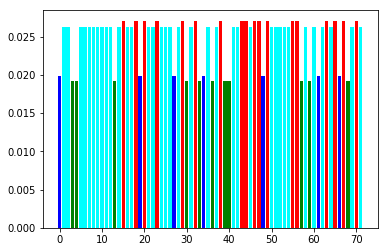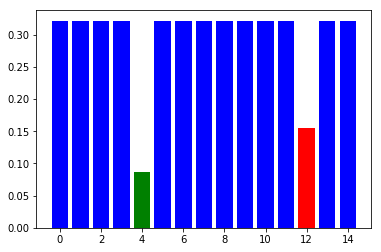We implement a Python Fusion model for the problem known as F-SPARC (fractional subcarrier and power allocation with rate constraints). Our implementation defines a mixed-integer exponential cone problem.

The model comes from the paper Bi-Perspective Functions for Mixed-Integer Fractional Programs with Indicator Variables by Adam N. Letchford, Qiang N and, Zhaoyu Zhong, http://www.optimization-online.org/DB_HTML/2017/09/6222.html.

Problem formulation¶

We consider a set $I$ of communication channels (subcarriers) to be assigned to a set $J$ of users. Each channel can be assigned to at most one user, while one user will typically have more channels assigned to them, subject to the requirement that user $j$ achieves a total data rate of at least $d_j$ (bits per second). The data rate of a channel is computed as follows: for a channel of bandwidth $B$ (hertz), noise level $N_i$ (watts) and operating with power $p_i$ (watts), the maximum data rate is $$f_i = B \log_2(1+\frac{p_i}{N_i}).$$ Finally, we assume a minimal system power of $\sigma$ required to keep the system running and a maximum power level $P$.

The goal is to map the channels to users and assign their power levels so that the energy efficiency

$$\frac{\textrm{total data rate}}{\textrm{total power}}$$

is maximized.

The mixed-integer formulation is then: $$\begin{array}{rl} \mathrm{maximize} & \frac{\sum_{i,j} B \log_2(1+p_{i,j}/N_i)}{\sigma+\sum_{i,j}p_{i,j}} \\ \textrm{subject to} & B \sum_i \log_2(1+p_{i,j}/N_i) \geq d_j, \quad j\in J, \\ & \sigma + \sum_{i,j}p_{ij} \leq P, \\ & 0 \leq p_{ij}\leq Px_{ij}, x_{ij}\in \{0,1\}, \\ & \sum_j x_{ij} \leq 1,\quad i\in I, \end{array}$$

where $p_{ij}$ is the power assigned to channel $i$ when used by user $j$. The first constraint describes the total bitrate of each user, the second bounds total power, and the remaining constraints with indicator variables model the assignment requirements.

Conic reformulation¶

To obtain a problem in conic form we perform a homogenization as in Section 5.2 of Letchfort et al. That is, we introduce new variables $t$, $z_{ij}$ and $\tilde{p_{ij}}$ with the intention of representing $$t = 1/(\sigma+\sum_{ij}p_{ij}),\quad z_{ij}=Bt\log_2(1+p_{ij}/N_i),\quad \tilde{p_{ij}} = p_{ij}t.$$ In terms of the new variables the problem is equivalent with

$$\begin{array}{rl} \mathrm{maximize} & \sum_{i,j} z_{ij} \\ \textrm{subject to} & z_{ij} \leq B t \log_2(1+\tilde{p_{i,j}}/(tN_i)), \quad i \in I, j\in J, \\ & d_jt \leq \sum_i z_{ij},\quad j\in J \\ & t\sigma + \sum_{i,j} \tilde{p_{i,j}} = 1, \\ & 1/P \leq t \leq 1/\sigma, \\ & 0 \leq \tilde{p_{i,j}} \leq x_{ij}, x_{ij}\in \{0,1\}, \\ & \sum_j x_{ij} \leq 1,\quad i\in I, \end{array}$$

The first constraint can equivalently be written as $$t+\tilde{p_{i,j}}/N_i \geq t \exp(\log(2)z_{i,j}/(Bt))$$ which, using the exponential cone, is $$(t+\tilde{p_{i,j}}/N_i , t, \log(2)z_{i,j}/B)\in K_\mathrm{exp}.$$

Implementation in Fusion¶

We start that functions which parse examples in the format of http://www.research.lancs.ac.uk/portal/en/datasets/ofdma-optimisation(48d843b0-1fb6-4e3d-af39-ca533a37b7ab).html and set up some global constants. The data files contain user demands and channel noise for each example.

In :
import ast, sys

constants = {
"System Power": 10,
"Bandwidth": 1.25,
"Maximum Power": 36
}

def parse(filename):
data = []
with open(filename, 'r') as file:
if 'Instance: ' not in content: raise Exception

for inst in content.split('Instance: ')[1:]:
if 'noise' not in inst or 'demand' not in inst: raise Exception

data.append({
"noise" : ast.literal_eval(inst.split('noise').split('demand').strip()),
"demand" : ast.literal_eval(inst.split('demand').strip())
})
return data

BW = constants["Bandwidth"]
P = constants["Maximum Power"]
SIGMA = constants["System Power"]

The next function is a direct implementation of the conic model above.

In :
from mosek.fusion import *
from math import log

def fsparc_model(n, d):
M = Model()
I, J = len(n), len(d)

# create variables
z = M.variable('z', [I, J], Domain.greaterThan(0.0))
x = M.variable('x', [I, J], Domain.binary())
p_tilde = M.variable('p_tilde', [I, J], Domain.greaterThan(0.0))
t = M.variable('t', 1, Domain.inRange(1.0/P, 1.0/SIGMA))

M.constraint(Expr.sum(x, 1), Domain.lessThan(1.0))
M.constraint(Expr.sub(p_tilde, x), Domain.lessThan(0.0))
M.constraint(Expr.sub(Expr.sum(z, 0), Expr.mulElm(Expr.repeat(t, J, 0), d)), Domain.greaterThan(0.0))

# objective
M.objective("obj", ObjectiveSense.Maximize, Expr.sum(z))

p_over_n = Expr.mulElm(p_tilde, [[1 / n_i] * J for n_i in n])
multi_t = Expr.repeat(Expr.repeat(t, I, 0), J, 1)
M.constraint(Expr.stack(2, Expr.add(multi_t, p_over_n), multi_t, Expr.mul(log(2.0) / BW, z)),
Domain.inPExpCone())

return M

Next we run the model and present some statistics for a few examples.

In :
%matplotlib inline
import matplotlib.pyplot as plt

def stats(M, n, d):
I, J = len(n), len(d)
t    = M.getVariable('t').level()
pval = M.getVariable('p_tilde').level().reshape([I, J]) / t
xval = M.getVariable('x').level().reshape([I, J])
power = [sum(pval[i,:]) for i in range(I)]
user = [int(sum([xval[i][j]*j for j in range(J)])) for i in range(I)]
print("Total data rate: demanded {0}, provided {1}".format(
sum(d),
sum([BW*log(1+power[i]/n[i])/log(2.0) for i in range(I)])))
print("Power: system {0:.3f}, used {1:.3f}, maximal: {2:.3f}".format(SIGMA, SIGMA+sum(power), P))
colors = ['red', 'green', 'blue', 'cyan', 'yellow', 'violet']
plt.bar(range(I), power, color = [colors[user[i]] for i in range(I)])

Example 1¶

We run an example with realistic data (72 channels, 4 users) with a very short time limit.

In :
instance = parse("data/lancaster/4_0.8.txt")
n, d = instance["noise"], instance["demand"]
M = fsparc_model(n, d)
M.setLogHandler(sys.stdout)
M.setSolverParam("optimizerMaxTime", 40.0)
M.solve()
M.acceptedSolutionStatus(AccSolutionStatus.Feasible)
stats(M, n, d)
Problem
Name                   :
Objective sense        : max
Type                   : CONIC (conic optimization problem)
Constraints            : 1229
Cones                  : 288
Scalar variables       : 1729
Matrix variables       : 0
Integer variables      : 288

Optimizer started.
Mixed integer optimizer started.
Presolve started.
Presolve terminated. Time = 0.01
Presolved problem: 1440 variables, 940 constraints, 2883 non-zeros
Presolved problem: 0 general integer, 288 binary, 1152 continuous
Clique table size: 72
BRANCHES RELAXS   ACT_NDS  DEPTH    BEST_INT_OBJ         BEST_RELAX_OBJ       REL_GAP(%)  TIME
0        1        1        0        NA                   3.2821073370e+02     NA          0.1
Cut generation started.
0        2        1        0        NA                   3.2821073370e+02     NA          0.6
Cut generation terminated. Time = 0.00
8        12       9        2        NA                   3.2564092596e+02     NA          1.0
21       25       22       5        NA                   3.2564092596e+02     NA          1.1
36       40       37       5        NA                   3.2564092596e+02     NA          1.2
49       53       50       6        NA                   3.2564092596e+02     NA          1.3
79       83       80       8        NA                   3.2564092596e+02     NA          1.5
159      163      160      12       NA                   3.2564092596e+02     NA          1.7
319      323      320      20       NA                   3.2564092596e+02     NA          2.3
639      643      640      36       NA                   3.2564092596e+02     NA          3.3
1079     1083     1080     58       NA                   3.2564092596e+02     NA          5.1
1540     1544     1519     4        9.6388958662e+01     3.2355936051e+02     235.68      7.0
2003     2003     1610     76       9.7146226251e+01     3.1948388561e+02     228.87      8.8
2483     2483     2056     10       9.7146226251e+01     3.1418591147e+02     223.42      10.4
2963     2963     2534     16       9.7146226251e+01     3.1198674503e+02     221.15      11.8
3442     3442     3011     40       9.7146226251e+01     3.1198674503e+02     221.15      13.4
3917     3917     3486     64       9.7146226251e+01     3.1198674503e+02     221.15      15.1
4389     4389     3870     13       9.7146226251e+01     3.1198474109e+02     221.15      17.0
4863     4863     4200     10       9.7146226251e+01     3.1068358231e+02     219.81      18.6
5343     5343     4680     11       9.7146226251e+01     3.0978236788e+02     218.88      20.1
5823     5823     5160     12       9.7146226251e+01     3.0943340745e+02     218.52      21.6
6303     6303     5624     9        9.7146226251e+01     3.0892256489e+02     218.00      23.2
6783     6783     6104     20       9.7146226251e+01     3.0848648896e+02     217.55      24.6
7260     7260     6581     44       9.7146226251e+01     3.0848648896e+02     217.55      26.1
7740     7740     7061     68       9.7146226251e+01     3.0848648896e+02     217.55      27.7
8209     8209     7522     12       9.7146226251e+01     3.0843778266e+02     217.50      29.5
8678     8678     7845     13       9.7146226251e+01     3.0824261215e+02     217.30      31.2
9156     9156     8281     14       9.7146226251e+01     3.0782433694e+02     216.87      33.0
9636     9636     8723     15       9.7146226251e+01     3.0758164100e+02     216.62      34.6
10115    10115    9152     16       9.7146226251e+01     3.0726996741e+02     216.30      36.2
10556    10556    9527     38       9.7146226251e+01     3.0726996741e+02     216.30      38.1
11034    11034    9989     62       9.7146226251e+01     3.0726996741e+02     216.30      39.7

Objective of best integer solution : 9.714622625080e+01
Best objective bound               : 3.072699674120e+02
Construct solution objective       : Not employed
Construct solution # roundings     : 0
User objective cut value           : 0
Number of cuts generated           : 0
Number of branches                 : 11034
Number of relaxations solved       : 11034
Number of interior point iterations: 242428
Number of simplex iterations       : 0
Time spend presolving the root     : 0.01
Time spend optimizing the root     : 0.09
Mixed integer optimizer terminated. Time: 40.03

Optimizer terminated. Time: 40.08

Integer solution solution summary
Problem status  : PRIMAL_FEASIBLE
Solution status : PRIMAL_FEASIBLE
Primal.  obj: 9.7146226251e+01    nrm: 8e+03    Viol.  con: 6e-05    var: 6e-10    cones: 0e+00    itg: 0e+00
Total data rate: demanded 1144.5349999999999, provided 1144.5614497476022
Power: system 10.000, used 11.782, maximal: 36.000The bar plot shows power assignments to the channels. Each color represents one user.

Example 2¶

The next example is a small, randomly generated set of data with 15 channels and 3 users, which we should solve to optimality.

In :
instance = parse("data/small-random/random_15_3_0.85.txt")
n, d = instance["noise"], instance["demand"]
M = fsparc_model(n, d)
M.setLogHandler(sys.stdout)
M.solve()
stats(M, n, d)
Problem
Name                   :
Objective sense        : max
Type                   : CONIC (conic optimization problem)
Constraints            : 199
Cones                  : 45
Scalar variables       : 271
Matrix variables       : 0
Integer variables      : 45

Optimizer started.
Mixed integer optimizer started.
Presolve started.
Presolve terminated. Time = 0.00
Presolved problem: 225 variables, 153 constraints, 452 non-zeros
Presolved problem: 0 general integer, 45 binary, 180 continuous
Clique table size: 15
BRANCHES RELAXS   ACT_NDS  DEPTH    BEST_INT_OBJ         BEST_RELAX_OBJ       REL_GAP(%)  TIME
0        1        1        0        NA                   6.2736906817e+01     NA          0.0
Cut generation started.
0        2        1        0        NA                   6.2736906817e+01     NA          0.1
Cut generation terminated. Time = 0.00
8        12       9        2        NA                   5.8418013107e+01     NA          0.2
20       24       21       5        NA                   5.7248034543e+01     NA          0.3
36       40       37       7        NA                   5.7248034543e+01     NA          0.3
48       52       49       8        NA                   5.7248034543e+01     NA          0.3
80       84       81       10       NA                   5.7248034543e+01     NA          0.4
160      164      161      14       NA                   5.7248034543e+01     NA          0.5
320      313      249      17       1.9890365163e+01     5.5150531676e+01     177.27      0.8
560      515      269      5        2.0074632174e+01     5.4015323987e+01     169.07      1.1
820      769      353      18       2.0886038578e+01     5.2713196601e+01     152.38      1.6
1160     1102     503      18       2.0886038578e+01     5.1086229306e+01     144.60      2.0
1619     1558     686      13       2.0886038578e+01     5.0036307959e+01     139.57      2.7
2081     2015     816      22       2.0886038578e+01     4.7777192266e+01     128.75      3.3
2545     2475     950      18       2.0886038578e+01     4.5168505100e+01     116.26      4.0
3008     2933     1043     21       2.0886038578e+01     4.2765322708e+01     104.76      4.6
3467     3391     1090     18       2.0886038578e+01     4.0000720055e+01     91.52       5.3
3913     3843     1186     21       2.0886038578e+01     3.8961479080e+01     86.54       6.0
4353     4292     1242     15       2.0886038578e+01     3.7279206008e+01     78.49       6.8
4803     4743     1316     9        2.0886038578e+01     3.6233564889e+01     73.48       7.5
5263     5200     1394     17       2.0886038578e+01     3.6162281699e+01     73.14       8.1
5717     5652     1446     21       2.0886038578e+01     3.5396585587e+01     69.47       8.8
6155     6097     1474     10       2.0886038578e+01     3.4835152140e+01     66.79       9.6
6574     6521     1477     19       2.0886038578e+01     3.4008845027e+01     62.83       10.4
7033     6977     1514     18       2.0886038578e+01     3.3563414586e+01     60.70       11.1
7474     7424     1523     14       2.0886038578e+01     3.3441191697e+01     60.11       11.9
7927     7879     1482     21       2.0886038578e+01     3.3056697831e+01     58.27       12.6
8376     8329     1467     18       2.0886038578e+01     3.2055350146e+01     53.48       13.2
8821     8777     1392     15       2.0886156731e+01     3.1721254007e+01     51.88       14.1
9275     9230     1338     14       2.0886156731e+01     3.1296712465e+01     49.84       14.8
9730     9684     1293     10       2.0886156731e+01     3.1084824213e+01     48.83       15.5
10172    10133    1255     16       2.0886156731e+01     3.0927284262e+01     48.08       16.2
10618    10580    1173     17       2.0886156731e+01     3.0531538141e+01     46.18       16.9
11060    11028    1089     14       2.0886156731e+01     3.0358038321e+01     45.35       17.7
11473    11455    1030     20       2.0886156731e+01     3.0272515219e+01     44.94       18.5
11898    11896    991      18       2.0886156731e+01     2.9876691828e+01     43.05       19.3
12327    12338    900      18       2.0886156731e+01     2.9590258263e+01     41.67       20.2
12743    12777    834      16       2.0886156731e+01     2.9590258263e+01     41.67       21.1
13180    13222    759      18       2.0886156731e+01     2.9590258263e+01     41.67       21.9
13600    13664    691      17       2.0886156731e+01     2.8856240125e+01     38.16       22.8
14033    14108    610      17       2.0886156731e+01     2.8758742060e+01     37.69       23.6
14483    14558    524      16       2.0886156731e+01     2.8140325871e+01     34.73       24.3
14918    14992    435      18       2.0886156731e+01     2.7798746872e+01     33.10       25.0
15335    15415    344      16       2.0886156731e+01     2.7511073832e+01     31.72       25.7
15675    15756    290      17       2.0886156731e+01     2.7223697972e+01     30.34       26.3
15955    16043    240      22       2.0886156731e+01     2.7223697972e+01     30.34       26.9
16195    16303    194      15       2.0886156731e+01     2.6706954318e+01     27.87       27.5
16375    16495    140      14       2.0886156731e+01     2.6240947330e+01     25.64       28.1
16515    16642    118      21       2.0886156731e+01     2.6240947330e+01     25.64       28.5
16614    16744    77       13       2.0886156731e+01     2.5988521964e+01     24.43       28.8
16673    16804    54       15       2.0886156731e+01     2.5988521964e+01     24.43       28.9
16713    16844    44       18       2.0886156731e+01     2.5988521964e+01     24.43       29.1
16750    16882    23       18       2.0886156731e+01     2.5988521964e+01     24.43       29.2
16761    16893    16       16       2.0886156731e+01     2.5988521964e+01     24.43       29.2
16776    16909    7        14       2.0886156731e+01     2.4433909459e+01     16.99       29.3
16786    16921    3        8        2.0886156731e+01     2.4433909459e+01     16.99       29.4
An optimal solution satisfying the relative gap tolerance of 1.00e-02(%) has been located.
The relative gap is 0.00e+00(%).
An optimal solution satisfying the absolute gap tolerance of 0.00e+00 has been located.
The absolute gap is 0.00e+00.

Objective of best integer solution : 2.088615673080e+01
Best objective bound               : 2.088615673080e+01
Construct solution objective       : Not employed
Construct solution # roundings     : 0
User objective cut value           : 0
Number of cuts generated           : 0
Number of branches                 : 16791
Number of relaxations solved       : 16927
Number of interior point iterations: 418244
Number of simplex iterations       : 0
Time spend presolving the root     : 0.00
Time spend optimizing the root     : 0.02
Mixed integer optimizer terminated. Time: 29.53

Optimizer terminated. Time: 29.55

Integer solution solution summary
Problem status  : PRIMAL_FEASIBLE
Solution status : INTEGER_OPTIMAL
Primal.  obj: 2.0886156731e+01    nrm: 6e+04    Viol.  con: 1e-05    var: 7e-10    cones: 0e+00    itg: 0e+00
Total data rate: demanded 297.7325530926529, provided 301.222514307648
Power: system 10.000, used 14.422, maximal: 36.000